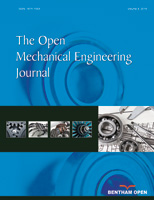# The Open Mechanical Engineering Journal

ISSN: 1874-155X ― Volume 13, 2019

# A Simple Mechanical Model for a Wiper Blade Sliding and Sticking Over a Windscreen

R. Barsotti, S. Bennati*, F. Quattrone
Division of Structural Engineering, Department of Civil and Industrial Engineering, University of Pisa, Italy

## Abstract

From the mechanical standpoint, wiper blades may be thought of as belonging to a category of systems in which some components are forced to slide with friction over each other or over some rough surface. Such systems, which are in widespread use in all areas of modern engineering, exhibit complex dynamic behavior, even when only a small number of degrees of freedom are involved. In this paper we reconsider a well-known, simple mechanical model in which a rigid block connected to a linear spring is free to slide over a rough surface. The surface moves according to a prescribed sinusoidal law. The model, despite its apparent simplicity, proves to be quite useful for studying the main dynamic features of such systems. In particular, herein the equations of motion are solved analytically and the exact sequence of sticking and sliding phases found. The influence on the solution of three dimensionless parameters chosen to describe the system is investigated, and some early indications provided on the set of possible long-term system responses. Lastly, a first evaluation of the different limit cycles for the block’s motion is illustrated.

Keywords: Driven oscillator, friction, limit cycles, stick-slip, wiper systems.

### Article Information

#### Identifiers and Pagination:

Year: 2016
Volume: 10
First Page: 51
Last Page: 65
Publisher Id: TOMEJ-10-51
DOI: 10.2174/1874155X01610010051

#### Article History:

Acceptance Date: 01/09/2015
Electronic publication date: 21/04/2016
Collection year: 2016

#### Article Metrics:

0

##### Total Statistics:

Full-Text HTML Views: 1089
Abstract HTML Views: 1005

##### Unique Statistics:

Full-Text HTML Views: 722
Abstract HTML Views: 622
Geographical View

open-access license: This is an open access article licensed under the terms of the Creative Commons Attribution-Non-Commercial 4.0 International Public License (CC BY-NC 4.0) (https://creativecommons.org/licenses/by-nc/4.0/legalcode), which permits unrestricted, non-commercial use, distribution and reproduction in any medium, provided the work is properly cited.

* Address correspondence to this author at the Division of Structural Engineering, Department of Civil and Industrial Engineering, University of Pisa, Largo Lazzarino 2, 56122 Pisa, Italy; Tel: +39 050 2218210; Fax: +39 050 2218201; E-mail: s.bennati@ing.unipi.it

## 1. INTRODUCTION

Windshield wiper systems are fundamental, albeit simple devices, nowadays mounted on all motor vehicles. Despite their simplicity, they play a key role in safety by ensuring that visibility is maintained at acceptable levels throughout a wide range of climatic and environmental conditions.

Under ordinary conditions wiper systems generally function flawlessly and relatively unobtrusively. However, in some particular cases undesired vibrations may develop and be disturbing for the driver, such as the chattering and squeaking that may occur, for example in the presence of dirt or dust deposited on the windshield, or for some values of the contact pressure between the rubber and the screen, or for some particular values of blade stiffness or windshield curvature.

Over recent decades, a number of studies have been conducted on the dynamics of wiper blades in order to improve or optimize their performance and investigate any conditions that may disrupt their proper, smooth functioning. In broad terms, the body of relevant literature on the topic may be subdivided into two main categories. The first category is represented by numerical studies, usually performed by means of finite element models of the entire wiping system or parts thereof (among the many examples, see Chevennement-Roux et al. [1C. Chevennement-Roux, T. Dreher, P. Alliot, E. Aubry, J-P. Lainé, and L. Jézéquel, "Flexible wiper system dynamic instabilities: modelling and experimental validation", Exp. Mech., vol. 47, pp. 201-210, 2007.
[http://dx.doi.org/10.1007/s11340-006-9027-3]
]. The second research approach, to which the present paper belongs, is conducted via simplified models which regard the wiping system or parts thereof as a mechanical system with a small number of degrees of freedom. The equations of motion can be solved analytically or numerically with much less computational effort than FEM analysis. With no aim to provide an exhaustive literature survey, we cite, by way of example, the 2DOF model representing the entire wiper system, including the two blades, described in Suzuki and Yasuda [2R. Suzuki, and K. Yasuda, "Analysis of chatter vibration in an automotive wiper assembly", JSME Int. J. Ser. C, vol. 41, no. 3, pp. 616-620, 1998.
[http://dx.doi.org/10.1299/jsmec.41.616]
], and that representing the rubber lip contained in Lencioni et al. [3G. Lancioni, S. Lenci, and U. Galvanetto, "Non-linear dynamics of a mechanical system with a frictional unilateral constraint", Int. J. Non-linear Mech., vol. 44, no. 6, pp. 658-674, 2009.
[http://dx.doi.org/10.1016/j.ijnonlinmec.2009.02.012]
].

In reality, simplified wiper system models are but a particular example of the mechanical models developed for the more general case of systems containing components that are forced to slide with friction over other components or

over an external surface, which may be either fixed or moving. Systems of the sort, which include brakes, turbines blades, digging buckets, and drill strings, exhibit quite complex dynamic behavior due to the nonlinearity induced by friction, even when only a small number of degrees of freedom are involved.

Over the last century particular attention has been devoted to the basic case of a single degree of freedom system subjected to friction forces. The first studies focusing on systems of this kind are contained in the fundamental work by Den Hartog [4J. Den Hartog, "Forced vibrations with combined coulomb and viscous friction", Trans. ASME, vol. 53, no. 9, pp. 107-115, 1931.], after which the same problem has been considered by many other researchers. Although the full list of contributions is too lengthy to cite here, some particularly relevant work on the issues at hand include, by way of example, the study of Parnes [5R. Parnes, "Response of an oscillator to a ground motion with coulomb friction slippage", J. Sound Vibrat., vol. 94, no. 4, pp. 469-482, 1984.], in which a single degree of freedom model is used to represent the contact between the soil and a buried pipe during a seismic event, the work of Marui and Kato [6E. Marui, and S. Kato, "Forced vibration of base-excited single-degree-of-freedom system with coulomb friction", J. Dyn. Syst. Meas. Control, vol. 106, no. 4, pp. 280-285, 1984.
[http://dx.doi.org/10.1115/1.3140686]
], which addresses the dynamics of an excited single degree of freedom system through the introduction of the “stopping region of motion”, that of Shaw [7S.W. Shaw, "On the dynamic response of a system with dry friction", J. Sound Vibrat., vol. 108, no. 2, pp. 305-325, 1986.
[http://dx.doi.org/10.1016/S0022-460X(86)80058-X]
] in which the results of Den Hartog are extended to the case that the static friction coefficient is different from the dynamic one, that of Hong and Liu [8H-K. Hong, and C-S. Liu, "Non-sticking oscillation formulae for coulomb friction under harmonic loading", J. Sound Vibrat., vol. 244, pp. 883-898, 2001.
[http://dx.doi.org/10.1006/jsvi.2001.3519]
], which illustrates some analytical solutions for a mass-spring system subjected to harmonic forces and free to slide over a fixed plane with friction, Andreaus and Casini [9V. Andreaus, and P. Casini, "Dynamics of friction oscillators excited by a moving base and/or driving force", J. Sound Vibrat., vol. 245, no. 4, pp. 685-699, 2001.
[http://dx.doi.org/10.1006/jsvi.2000.3555]
], which instead analyzes the dynamics of a friction oscillator subjected to both ground base motion and a driving force and, more recently, Gibert et al. [10J.M. Gibert, G. Fadel, and M.F. Daqaq, "On the stick-slip dynamics in ultrasonic additive manufacturing", J. Sound Vibrat., vol. 332, no. 19, pp. 4680-4695, 2013.
[http://dx.doi.org/10.1016/j.jsv.2013.04.007]
], which analyses the stick-slip dynamics in ultrasonic additive manufacturing, and Butikov [11E.I. Butikov, "Spring pendulum with dry and viscous damping", Commun. Nonlinear Sci. Numer. Simul., vol. 20, no. 1, pp. 298-315, 2015.
[http://dx.doi.org/10.1016/j.cnsns.2014.04.026]
], which studies the free and forced oscillations of a torsion spring pendulum damped by viscous and dry friction.

The present work reconsiders a well-known simple model that enables providing an elementary description of the dynamic response of systems like those described in the foregoing. The model, which is illustrated in section 2, regards a rigid block connected to an elastic spring; the block is free to slide over a rough surface that moves according to a sinusoidal law. A suitably modified version of Coulomb’s friction law describes the contact between the block and the underlying surface.

A description of the different types of motion that the system can undergo is provided in section 3. We focus our attention on the role played by three dimensionless parameters: the ratio between the oscillation frequency of the surface and the natural frequency of the mass-spring system; the ratio between the kinetic and static friction coefficients, and the ratio between the maximum amplitude of the surface oscillations and the maximum distance of the block from the origin, which would correspond to it being in a state of static equilibrium (if the surface were motionless). The same section illustrates an example application regarding a wiper blade cross-section.

Lastly, in section 4, we investigate some of the different limit cycles - corresponding to continuous sticking or sliding - that the system may actually undergo. We aim at showing that once the main system parameters are fixed, it is possible to foresee some features of the possible long term responses. In this regard, by using the simple model described herein, we draw up a map that seems able to provide useful indications on potential long term responses of the system. The preliminary results deduced in this way, although by no means exhaustive, can then be compared with some of the analytical and numerical results available in the literature.

## 2. THE MECHANICAL MODEL

The mechanical problem addressed here concerns the motion of the wiper blade shown schematically in Fig. (1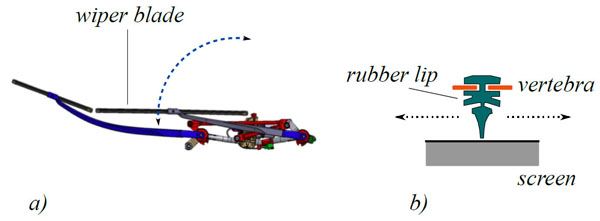). The wiper blade, which is the component of the wiper system that actually sweeps across the windshield in motor vehicles, is composed of a slender metallic element (the vertebra) in contact with the underlying surface (the screen) through a very deformable rubber layer. The wiper blade is connected to a metallic beam (the arm), which is in turn connected to an electric engine that provides the torque needed to move the blade across the screen.Fig. (1)(a) schematic representation of a wiper system; (b) cross section of the wiper blade.

Finding the exact solution to the aforementioned dynamic problem is quite difficult. The blade is an elastic element with an infinite number of degrees of freedom, and it undergoes large displacements. Moreover, a nonlinear visco-elastic layer is in contact with the screen. Lastly, friction and unilateral contact conditions add two more relevant nonlinearities to the problem.

Here, without searching for the exact solution to this problem, we aim to investigate some of the main features of the dynamic response of a wiper blade by means of a simple model. To this end, let us consider the single degree-of-freedom system represented in Fig. (2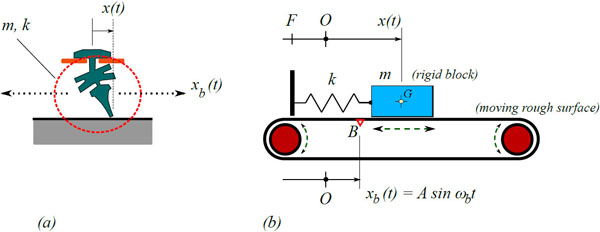), which has been the object of some preliminary studies by the authors in [12R. Barsotti, S. Bennati, and F. Quattrone, "Dyanamical response of a single degree of freedom system in contact with a rough moving surface. (in Italian)", G. Lacidogna, and A. Carpinteri, Eds., Proceedings of AIMETA, 2013 ]. Here, the wiper blade is schematized, as a first rough approximation, as a rigid block with mass m attached to a spring with elastic constant k. The other end of the spring is connected to a given fixed point F. The block is pushed down in contact with an underlying rigid rough surface by a force P. We choose to describe the system’s motion from the perspective of an observer on the vertebra. Thus, in order to account for the relative motion between the wiper blade and the screen, we assume that the underlying surface is moving according to a prescribed law. We set the origin of our reference system at point O, where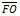is the initial length of the unstrained spring. Lastly, we indicate by x(t) the position of the block’s center of gravity with respect to O.Fig. (2)(a) Wiper blade cross section; (b) The corresponding single degree of freedom system.

On the moving surface, we consider point B, located directly beneath the origin O at t = 0. We assume that at any time the position, xb, of this point is described by the harmonic law xb = Asinωbt, where A (for simplicity’s sake assumed to be positive) and ωb are the amplitude and the angular frequency of the surface’s motion, respectively.

### 2.1. A Modified Version of Coulomb’s Friction Law

The friction law used in this model is a suitably modified version of Coulomb’s original formulation. As is customary, the static and dynamic friction coefficients are indicated by µs and µk, respectively. The friction force applied to the block by the surface, Fa, is determined according to the following relations. Denoting rel = -b as the relative velocity between the block and the surface, we set Fa = -Fkrel / |rel|, where Fk = µk (mg + P), when rel ≠ 0 (g is the acceleration of gravity), while |Fa| ≤ Fs = µs (mg + P) if the block is sticking to the surface.

From a strictly formal point of view, according to the standard version of Coulomb’s law, as soon as a sliding phase ends, the magnitude of the friction force |Fa| could raise instantaneously up to the maximum static value Fs from the very first instant of sticking. This is naturally an idealization. In actual fact, when the block stops sliding, i.e., from the moment rel = 0, it will take a (usually very) short time to reestablish contact conditions, which correspond to static friction between the block and the surface (in this regard, see, for example, the experimental studies of Sampson et al. [13J. Sampson, F. Morgan, D.W. Reed, and M. Muskat, "Friction behavior during the slip portion of the stick-slip process", J. Appl. Phys., vol. 14, no. 12, pp. 689-700, 1943.
[http://dx.doi.org/10.1063/1.1714948]
]. Thus, accounting for this aspect calls for allowing for a gradual change in the friction force from the dynamic to the static value at the beginning of each sticking phase of the block.

Now, in order to account for the aforementioned feature in a relatively simple, approximate way, we assume that the friction force obeys the following rules:

• in any sliding phase, the friction force is equal to the kinematic value;
• at the beginning of any sticking phase, the friction force cannot exceed the kinematic value;
• at any given time within any sticking phase, the friction force cannot exceed the static value.

In other words, at any given time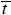, the adopted friction law may be expressed as: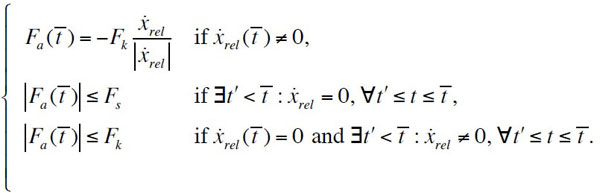(1)

As will be shown in the following, relation (1) enables deciding whether a new sticking phase for the block may actually start during its motion. To this end, we determine the value of the friction force, Fa, that should be exerted on the block under sticking condition, and we check whether the necessary condition |Fa| < Fk is fulfilled at the onset of the sticking phase (a more detailed explanation of this is given in section 3.2).

## 3. DESCRIPTION OF THE MOTION

In the following, we assume that observation of the block’s motion begins at a given initial time t0 . We indicate by x0 = x(t0 ) and 0 = (t0 ) the initial position and velocity, respectively. In the general case, the motion of the system will be made up of a sequence of sticking phases, during which the block follows the motion of the surface, and sliding phases, during which the mass moves with respect to the surface. From a formal point of view, the equation of motion may be written as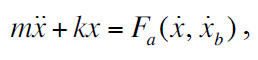(2)

where Fa is the non-smooth friction force according to (1). Broadly speaking, any sticking phase following a sliding phase will begin at a given timeif both the block and the surface have the same velocity, and if the magnitude of the friction force that the surface exerts on the block at t =is lower than the kinetic friction force, i.e., if |Fa ()| < Fk. Depending on the values of the main system parameters, the sticking phase may last indefinitely. In such case, |Fa| will remain lower than the maximum static value Fs for any t.

Vice versa, any sliding phase following a sticking phase may begin at a given time t =only if the magnitude of the reactive force that the surface exerts on the block reaches the maximum static friction value, i.e., if |Fa ()| = Fk. Sliding of the block actually begins if a friction force larger than Fs would be required to ensure sticking for t >. Under appropriate conditions, the sliding phase may also last indefinitely. Nevertheless, when a sliding phase ends, the relative velocity between the block and the surface is nil. In the next sections, both the sticking and the sliding phases will be analyzed in detail, and particular attention will be dedicated to some of the limit cycles corresponding to continuous sliding or sticking.

It is worth noting that, by performing the change of variable

 xrel (t) = x (t) - xb (t),

the equation of motion becomes equivalent to that of the long-studied, well-known mechanical system addressed by Den Hartog [4J. Den Hartog, "Forced vibrations with combined coulomb and viscous friction", Trans. ASME, vol. 53, no. 9, pp. 107-115, 1931.] and, among many others, Shaw [7S.W. Shaw, "On the dynamic response of a system with dry friction", J. Sound Vibrat., vol. 108, no. 2, pp. 305-325, 1986.
[http://dx.doi.org/10.1016/S0022-460X(86)80058-X]
], Hong and Liu [8H-K. Hong, and C-S. Liu, "Non-sticking oscillation formulae for coulomb friction under harmonic loading", J. Sound Vibrat., vol. 244, pp. 883-898, 2001.
[http://dx.doi.org/10.1006/jsvi.2001.3519]
], Csernák and Stépán [14G. Csernák, and G. Stépán, "On the periodic response of a harmonically excited dry friction oscillator", J. Sound Vibrat., vol. 295, no. 3-5, pp. 649-658, 2006.
[http://dx.doi.org/10.1016/j.jsv.2006.01.030]
], composed of a block subjected to a harmonic load and free to slide with friction over a rigid fixed surface. The equation of motion for such a system reads [10J.M. Gibert, G. Fadel, and M.F. Daqaq, "On the stick-slip dynamics in ultrasonic additive manufacturing", J. Sound Vibrat., vol. 332, no. 19, pp. 4680-4695, 2013.
[http://dx.doi.org/10.1016/j.jsv.2013.04.007]
]

 mẍrel + kxrel = Fa (ẍrel) - po sin (ωb t), (3)

 po = mAω2 (1 - Ω2), (4)

and whereis the natural frequency of the system, while Ω = ωb / ω is the frequency ratio. For given values of A, ω and ωb, the above relation enables assessing the corresponding value of p 0.

Although the two alternative mathematical descriptions are equivalent, there are at least two reasons for preferring (2) over (3) as the equation of motion for the mechanical model of the wiper blade. The first is a matter of convenience: if equation (3) is used, the magnitude of the equivalent harmonic load (4) depends on both the amplitude and the frequency of the surface oscillations. Thus, the two main parameters describing the input in our system would be intermingled. This observation suggests that direct study of the dynamics of the system we aim to investigate, in which the block is set down in contact with an underlying moving surface, is facilitated by using (2) rather than (3).

The second, but no less important, reason is that relation (4) does not represent a one-to-one correspondence in the relevant case in which Ω = 1. In such a case, in fact, the amplitude p 0 of the equivalent harmonic load would be equal to zero irrespective of the amplitude A of the surface oscillations. Consequently, by using the equivalent harmonically loaded system (3), the relevant phenomenon called resonant locking (i.e., the mass oscillates as if it were attached to the surface), recently highlighted by Chatterjee [15S. Chatterjee, "Resonant locking in viscous and dry friction damper kinematically driving mechanical oscillators", J. Sound Vibrat., vol. 332, no. 14, pp. 3499-3516, 2013.
[http://dx.doi.org/10.1016/j.jsv.2012.12.042]
], would be hindered. On the contrary, such phenomenon emerges clearly by considering the surface motion directly, as we have chosen to do.

In other terms, the two alternative formulations of the problem at hand, although formally equivalent, are not equivalent in terms of the clarity and readability of the results obtainable. We will return on this issue later on.

### 3.1. The Sticking Phase

Let us assume that the block starts sticking to the surface, and let us indicate with t1 the start time of the sticking phase. At t = t1, the relative velocity between the block and the surface is zero, and the law of motion for the block during the sticking phase is:

 x (t) = A sin (ωb t) + C, t ≥ t1, (5)

where C is the constant relative position between the block and point B of the surface.

The block will stick to the surface until the magnitude of the force exchanged between it and the surface reaches the maximum static friction value, Fs. This condition is expressed by:

 |mẍ (t) + kx (t)| ≤ Fs, (6)

from which, by making use of (5), we obtain:

 |α (1 - Ω2) sinωb t + λ| ≤ 1, (7)

where α = A / xs, λ = C / xs, xs = Fs / k, and Ω =ωb / ω is the frequency ratio, in whichis the natural frequency of the mass-spring system. Equation (7) allows for determining the pairs (α, Ω) and the values of the dimensionless initial position of the block, λ, which correspond to a block sticking to the surface during its entire motion. We thus obtain: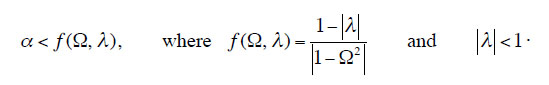(8)

In graphical terms, this condition corresponds to the region of the three-dimensional space (α, λ, Ω) bounded from above by the limit surface α = f (Ω, λ).

The points beneath the surface, whose coordinates satisfy (8), represent those systems whose motion will be characterized by continuous sticking for tt1. Conversely, the points above the surface represent those systems that will, sooner or later, undergo at least one sliding phase. Lastly, the points belonging to the surface are bifurcation points corresponding to systems for which relative motions of different kind are possible. In this case, the block may either keep sticking to the surface indefinitely or going through a sequence of sliding and sticking phases.

It is a simple matter to check that the maximum value for α that satisfies (8) for fixed Ω is obtained for λ = 0. Thus, in the (Ω, α) plane, the curve α = 1 / |1 - Ω2| = f 0(Ω) (Fig. 3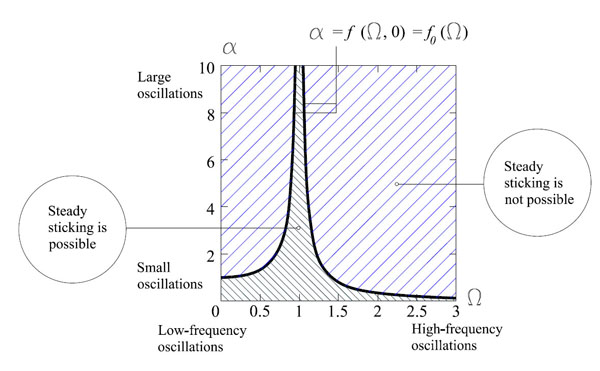) enables distinguishing the systems for which the block may undergo endless sticking motion (region under the curve) from those systems for which such motion is impossible. More precisely, when the point with coordinates α and Ω is beneath the curve, i.e.,

 α < fo (Ω), (9)

there exists at least one set of initial conditions such that the block keeps sticking from the beginning; contrariwise, when the point is above the curve, the block will undergo at least one sliding phase during its motion.

It is worth noting that for given values of α and λ (with |λ| < 1), if Ω belongs to the interval comprised between the values: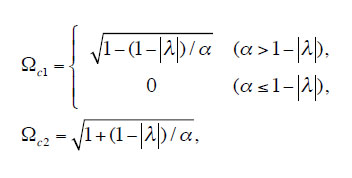(10)

(i.e., if the condition Ωc1 ≤ Ω ≤ Ωc2 is satisfied), motion without any slippage becomes possible for the block. In the particular case in which Ω = 1, this kind of motion is possible regardless of the value of α. Thus, if the system’s natural frequency and that of the surface oscillations are equal, there is at least one set of initial conditions for which the block sticks to the surface as if it were attached to it, regardless of the amplitude of the oscillations. This is what Chatterjee [15S. Chatterjee, "Resonant locking in viscous and dry friction damper kinematically driving mechanical oscillators", J. Sound Vibrat., vol. 332, no. 14, pp. 3499-3516, 2013.
[http://dx.doi.org/10.1016/j.jsv.2012.12.042]
] has termed resonant locking.

Whenever the force that the surface exerts on the block reaches the maximum static friction value, a sliding phase may take place. In these cases, by using eqn. (6), we can evaluate the corresponding time, t2, by solving: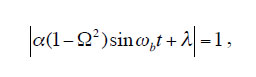(11)

This equation holds regardless of the initial time, t1, of the sticking phase, and has two solutions: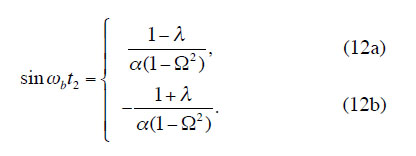(12a,b)

It can be proved that of the two solutions, the appropriate one to consider will depend on the value of α |1 - Ω2|, and the sign of cosωbt1 (details are given in Appendix A). In other words, the proper end time, t2, for the generic sticking phase can be determined according to the following sticking end-time criterion.

• If λ = 0, then

- if (1 - Ω2) cosωbt1 > 0, t2 is determined according to (12a);

- if (1 - Ω2) cosωbt1 < 0, t2 is determined according to (12b).

• If λ > 0 and if α|1 - Ω2| < 1 + λ, t2 is determined according to (12a).

• If λ > 0 and if α|1 - Ω2| ≥ 1 + λ, then

- if (1 - Ω2) cosωbt1 > 0, t2 is determined according to (12a);

- if (1 - Ω2) cosωbt1 < 0, t2 is determined according to (12b).

• If λ < 0 and if α|1 - Ω2| < 1 - λ, t2 is determined according to (12b).

• If λ < 0 and if α|1 - Ω2| ≥ 1 - λ, then

- if (1 - Ω2) cosωbt1 > 0, t2 is determined according to (12a);

- if (1 - Ω2) cosωbt1 < 0, t2 is determined according to (12b).

### 3.2. The Sliding Phase

Let us assume that at some given instant t = t3 the block is sliding over the surface. The block’s equation of motion in the sliding phase reads:

 mẍ + kx = ±Fk. (13)

where the sign depends on that of the relative velocity, rel = -b. during the sliding phase. Integration of Equation. (13) yields the law of motion:

 x (t) = c1 cosωt + c2 sinωt ± xk. (14)

where xk = ηxs, and η = µk / µs.Fig. (3)The (Ω, α) regions where endless sticking is possible and where it is not.

In many cases, however, the sliding phase of the block’s motion starts at the end of a sticking phase. In this situation, the start time of the sliding phase coincides with the solution to (12a) or (12b), i.e. t3 = t2, and the relative velocity at the beginning of the sliding phase is zero, rel(t3) = 0. Thus, the sign of the friction force is undetermined, and a criterion for determining the sign of the force at the onset of the sliding phase is needed. It is a relatively simple matter to verify that (relative velocity sign criterion):

• If t2 is determined according to (12a), the relative velocity at the onset of sliding will be negative, and the right-hand side of (13) is +Fk;
• If t2 is determined according to (12b), the relative velocity will be positive, and the right-hand side of (13) is -Fk.

The necessary condition for a sliding phase to stop at instant t4 is that the relative velocity between the block and the surface become zero, (t4) = b(t4). However, a sticking phase may actually begin at instant t4 only if the additional condition |mẍ (t4) + kx(t4)| ≤ Fk is fulfilled, that is if:

 | -αΩ2 sin (ωb t4) + x4 / xs| ≤ η ≤ 1, (15)

where x4 = x(t4), which ensures that at the beginning of a sticking phase the magnitude of the force exchanged between the block and the surface is lower than the kinematic friction force, Fk.

If relation (15) is not satisfied, the force that the surface exerts on the block at t = t4 would be greater than Fk, or at least equal to it. In this case, a sticking phase cannot begin, and the block will continue sliding over the surface.

## 4. SOME REMARKS ABOUT LIMIT CYCLES

Limit cycles are periodic solutions to the equation of motion characterized by a net balance between the amounts of energy dissipated by and supplied to the mass-spring system over each period. Different types of limit cycles may be observed for systems similar to those considered here ([8H-K. Hong, and C-S. Liu, "Non-sticking oscillation formulae for coulomb friction under harmonic loading", J. Sound Vibrat., vol. 244, pp. 883-898, 2001.
[http://dx.doi.org/10.1006/jsvi.2001.3519]
], [16G. Licskó, and G. Csernák, "On the chaotic behaviour of a simple dry-friction oscillator", Math. Comput. Simul., vol. 95, pp. 55-62, 2014.
[http://dx.doi.org/10.1016/j.matcom.2013.03.002]
]).

By following the evolution of the system starting from different initial conditions, and by considering different values of the main system parameters (i.e., α, Ω and η), it can be seen that the trajectories tend towards a periodic trend. In particular, after an initial transient phase, they approach limit cycles, where the block keeps sliding indefinitely, others where it keeps sticking, and yet others where it alternately slides and stick. In this section, we concentrate our attention on the limit cycles of the same period as the surface’s oscillations, corresponding to pure sliding and pure sticking.

### 4.1. Sticking Limit Cycles

It is straightforward to conclude that, if sticking condition (9) is fulfilled, periodic solutions to the equation of motion characterized by continuous sticking to the surface become possible. All these periodic solutions may be expressed as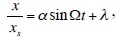(16)

where the λ parameter represents the dimensionless initial position of the block, which in turn must verify the limitation

 |λ| ≤ 1 - α |1 - Ω2|. (17)

It is worth observing that, when α = 1 / |1 - Ω2|, λ = 0 is the only value that satisfies (17), and the corresponding systems are in a limit condition.

The continuous sticking periodic solutions illustrated above may also represent possible limit cycles (if any) for systems whose parameters α and Ω do fulfill condition (9). Nevertheless, it cannot be excluded a priori that other limit cycles, for example limit cycles represented by alternating sticking and sliding phases, may become possible for such systems. Actually, stick-slip limit cycles may be numerically determined by suitably fine-tuning the system’s parameters. We will return to this point in the following, where we will show that by choosing suitable values of α, Ω and η, the same system may even exhibit different limit cycles depending on the particular initial conditions.

### 4.2. Sliding Limit Cycles

Let us consider a block that by hypothesis is undergoing periodic motion. Let us assume that the block slides over the surface, without ever sticking to it, and that the period of motion coincides with that of the oscillating surface, Tb = 2π / ωb. According to the results of [17M. Pascal, "New limit cycles of dry friction oscillators under harmonic load", Nonlinear Dyn., vol. 70, no. 2, pp. 1435-1443, 2012.
[http://dx.doi.org/10.1007/s11071-012-0545-5]
], it is straightforward to conclude that the block’s trajectory in the (t, x) plane is symmetrical, except for the particular cases corresponding to Ω = 1 / n (n, 2,3, ). Thus, we restrict our attention to symmetrical motion with respect to the point (Tb / 2, 0) on the time axis, at which the block oscillates around the origin. Restricting our attention to the single period covering the time interval [0, Tb], we set:

 x (t) = -x (t + Tb / 2), x (0) = x (Tb / 2) = x (Tb) = 0. (18)

Moreover, with reference to the same time interval, we write the law of motion of the surface as xb(t) = Asin(ωbt + ϕ), where the angle ϕ accounts for the phase shift between the harmonic oscillation of the block and the surface.

Due to the assumed symmetry properties of the trajectory, the component of the friction force in the direction of the x axis will be equal to +Fk over one half of the period and to -Fk over the other half. By accounting for conditions (18) and by referring to Eqn. (14), the block’s trajectory in the time interval during which, for example, the friction force component is positive, can be expressed as: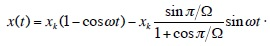(19)

An analogous expression for the other half period (where the friction force component is equal to -Fk) can be easily obtained.

We can further determine the phase angle ϕ by imposing that the velocity of the surface be equal to that of the block at the beginning of any half-period (i.e., by setting rel(0) = rel(Tb / 2) = rel(Tb) = 0), thus yielding: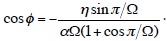(20)

The periodic motion described by Eqn. (19) may actually take place if the phase angle ϕ satisfies (20). Moreover, at the beginning of any half-period, when the relative velocity is zero, the sticking condition (15) must not be fulfilled. Therefore, by taking into account that for t = 0, Tb / 2, Tb…, the position occupied by the block is x = 0, we obtain the following additional condition for the validity of the periodic solution we are considering: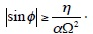(21)

By using both (20) and (21), we can eliminate the phase angle ϕ. Thus, it is possible to reduce to one single condition expressed in terms of the system parameters α, Ω and η:(22)

Expressions analogous to (22) have been obtained by Hong and Liu [8H-K. Hong, and C-S. Liu, "Non-sticking oscillation formulae for coulomb friction under harmonic loading", J. Sound Vibrat., vol. 244, pp. 883-898, 2001.
[http://dx.doi.org/10.1006/jsvi.2001.3519]
] and Csernák and Stépán [14G. Csernák, and G. Stépán, "On the periodic response of a harmonically excited dry friction oscillator", J. Sound Vibrat., vol. 295, no. 3-5, pp. 649-658, 2006.
[http://dx.doi.org/10.1016/j.jsv.2006.01.030]
] by considering a harmonically loaded system free to slide over a fixed rough surface, although minor differences can be recognized between the friction law accounted for in these works and that introduced here. Lastly, as was recently evidenced by Butikov [11E.I. Butikov, "Spring pendulum with dry and viscous damping", Commun. Nonlinear Sci. Numer. Simul., vol. 20, no. 1, pp. 298-315, 2015.
[http://dx.doi.org/10.1016/j.cnsns.2014.04.026]
], expression (20) is not valid if 1 / Ω is an odd integer, while an infinite number of non-symmetrical limit cycles, plus the symmetrical one, are possible in the particular case in which 1 / Ω is an even integer.

### 4.3. Limit Cycles a Given System May Attain: Some Considerations

As has already been pointed out, the motion of the system can be determined if the adopted values of the system’s main parameters, α, Ω and η, are chosen, together with some given initial conditions. Consequently, it is reasonable to assume that setting the values of said parameters should also enable determining the long-term response of the system. In other words, if α, Ω and η were known, it should be possible to predict the system’s evolution and the existence of some limit cycle to which the system potentially tends as well.

Here, with no claim to providing a full solution to the issue described above, we wish to demonstrate that the two inequalities (9) and (22) enable making some predictions about the periodic solutions (i.e., continuous sliding, continuous sticking, alternating sticking and sliding) that may actually be observed in a given system. That is, we will show that if the parameters describing the system, α, Ω and η, are assigned, it is possible to forecast some features of its corresponding long-term response.

For given η, the limitations expressed by the aforementioned two inequalities can be effectively represented in the (Ω, α) plane. By way of example, Fig. (4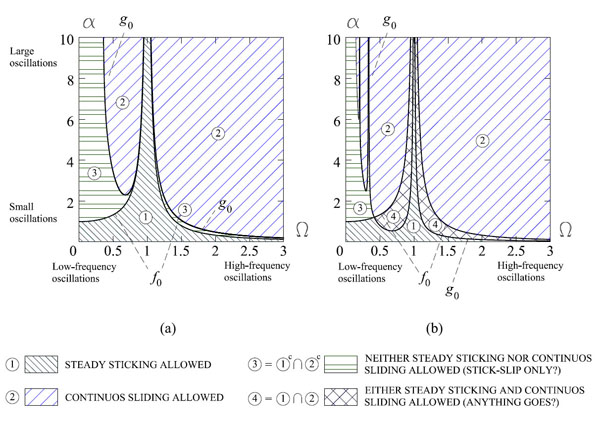) shows the partition of the (Ω, α) plane generated by inequalities (9) and (22) for η = 0.85 and η = 0.2, respectively. Our analysis will be limited to sliding, sticking, and stick-slip limit cycles.

The points whose coordinates satisfy (22) are those above the g 0 curve. Instead, the points below the f 0 curve, already represented in Fig. (3), correspond to the systems that allow continuous sticking, according to inequality (9). The two sets of points may be disjoined (Fig. 4a) or not (Fig. 4b), depending on the value of η. More precisely, such an intersection is not empty when η falls below the threshold value= 0.8264.

Each region drawn in Fig. (4) corresponds to different possible long-term responses for the system. In particular, if the point corresponding to a given system belongs to region 1, steady sticking is allowed, but continuous sliding is not possible at all whatever the initial conditions may be. On the contrary, continuous sliding is allowed (but steady sticking is not) in region 2.

In the systems whose corresponding points fall in region 4, that is, at the intersection between 1 and 2 (in case it is not empty), both steady sticking and continuous sliding become possible. For such systems, the specific initial conditions determine the kind of long term response the system actually undergoes. On the contrary, in region 3, external to both region 1 and 2, neither the continuous sliding nor the continuous sticking periodic solution is reachable for the system. In the latter case, limit cycles comprised of an alternating sticking and sliding phases are without doubt possible.

The large number of numerical simulations performed, omitted here for the sake of brevity, seem to confirm such predictions. It is worth stressing that the analyses performed so far cannot exclude the presence of stick-slip periodic solutions in the other three regions as well. Thus, within region 4, no conclusions can be drawn from the present analysis.Fig. (4)Partition of the (Ω, α) plane showing possible long-term system responses for (a) η=0.85, and (b) η=0.2.

It should be noted in passing that the overall picture represented in Fig. (4) could not have been obtained if the system had been studied via the equivalent harmonic load technique. One main novelty of the present study, as already noted in section 3, consists in its highlighting the resonance locking phenomenon, which is made clearly visible by directly accounting for the surface oscillations. In this regard, it is enough to point out that when Ω is equal to unity, the block sticks to the surface regardless of the value of α. Thus, in such case the amplitude of the oscillations of the block is not infinite, as may be erroneously concluded using some approaches based on harmonically loaded systems, but coincides with that of the surface.

It is interesting to note that the partition of the (Ω, α) plane described above appears to be in full agreement with some numerical results on harmonically loaded systems, such as those illustrated in [14G. Csernák, and G. Stépán, "On the periodic response of a harmonically excited dry friction oscillator", J. Sound Vibrat., vol. 295, no. 3-5, pp. 649-658, 2006.
[http://dx.doi.org/10.1016/j.jsv.2006.01.030]
], where stick-slip motion is revealed numerically when the magnitude of the harmonic load is increased above some threshold value. The appearance of such stick-slip motion may be easily and exactly predicted for within the same range of parameter values, with no need to resort to numerical solutions, by simply checking whether the representative point of the system lies within region 3 of Fig. (4) or not.

Direct analysis of the system free to slide over a moving surface also furnishes a simple explanation for the progressive growth of the number of sticking phases as Ω goes to zero. It is a simple matter to see that, in such a case, the surface motion tends towards uniform linear motion with constant velocity, and therefore the system approaches the behavior of a simple stick-slip oscillator [18E. Rabinowicz, "The intrinsic variables a_ecting the stick-slip process", Proc. Phys. Soc., vol. 71, no. 4, pp. 668-675, 1958.
[http://dx.doi.org/10.1088/0370-1328/71/4/316]
].

It is worth noting that for small η (Fig. 4a), region 3 on the right-hand side of the (Ω, α) domain disappears. Moreover, region 4 appears (i.e., there are systems that may evolve towards any one of the three possible kinds of responses), and the border between the slip and stick-slip regions for Ω < 1 becomes more jagged. This might suggest that chaotic responses are likely to be observed in such cases, a suggestion that is in very good agreement with the results obtained by Gibert et al. [10J.M. Gibert, G. Fadel, and M.F. Daqaq, "On the stick-slip dynamics in ultrasonic additive manufacturing", J. Sound Vibrat., vol. 332, no. 19, pp. 4680-4695, 2013.
[http://dx.doi.org/10.1016/j.jsv.2013.04.007]
].

Clearly the above-illustrated appraisal of the system’s long-term response is by no means conclusive. Further studies are needed to carry out an exhaustive examination of all possible periodic solutions and their corresponding limitations as well. Nevertheless, the first results illustrated here seem promising, and the partition of the (Ω, α) plane, though still requiring completion, provides some first basic indications.

## 5. AN APPLICATION EXAMPLE: THE POSSIBLE MOTION OF A WIPER BLADE

The literature contains quite a large body of works proposing either numerical or semi-analytical solutions to the equation of motion of systems like those considered here. Numerical solution procedures are widely adopted and reported on. By way of example, we cite the works of Leine et al. [19R.I. Leine, D.H. Van Campen, and A. De Kraker, "Stick-slip vibrations induced by alternate friction models", Nonlinear Dyn., vol. 16, pp. 41-54, 1998.
[http://dx.doi.org/10.1023/A:1008289604683]
], and Stein et al. [20G.J. Stein, R. Zahoranský, and P. Múčka, "On dry friction modelling and simulation in kinematically excited oscillatory systems", J. Sound Vibrat., vol. 311, pp. 74-96, 2008.
[http://dx.doi.org/10.1016/j.jsv.2007.08.017]
], which follow different approaches to simulating single-degree-of-freedom systems subjected to friction.

The alternative strategy, which we follow here, is represented by semi-analytical solutions. It consists of assembling the analytical expressions for the equations of motion that hold in the sticking and the sliding phases. Examples of such a solution strategy can be found in the works by Shaw [7S.W. Shaw, "On the dynamic response of a system with dry friction", J. Sound Vibrat., vol. 108, no. 2, pp. 305-325, 1986.
[http://dx.doi.org/10.1016/S0022-460X(86)80058-X]
], and more recently, Gibert et al. [10J.M. Gibert, G. Fadel, and M.F. Daqaq, "On the stick-slip dynamics in ultrasonic additive manufacturing", J. Sound Vibrat., vol. 332, no. 19, pp. 4680-4695, 2013.
[http://dx.doi.org/10.1016/j.jsv.2013.04.007]
], amongst others.

To illustrate our solution method, let us consider by way of example the start time of any given sticking phase (point a, Fig. 5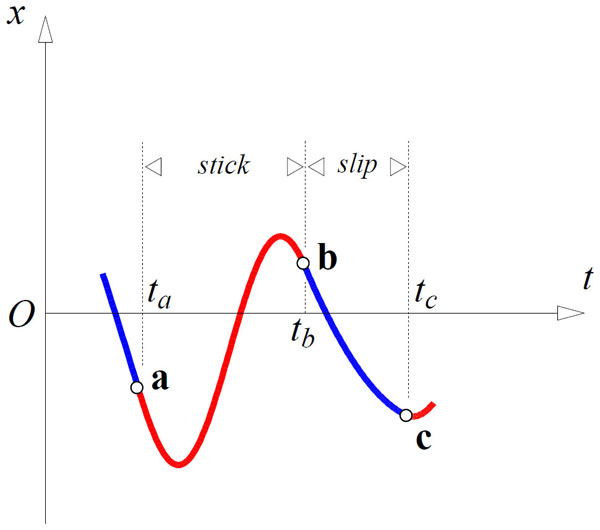). If the block alternatively slides and sticks, the solution procedure is organized as follows (if the block slides or sticks indefinitely, the modifications to the procedure are straightforward).

1. We determine the start time of the next slip phase, tb, corresponding to point b by means of Eqn. (12a) or (12b)
2. In the [ta, tb] interval, we describe the (sticking) motion via Eqn. (5).
3. We determine the direction of the friction force at time tb by using the relative velocity sign criterion.
4. We determine the time tc corresponding to point c by solving the transcendent equation rel = 0, and check condition (15).
5. In the [tb, tc] interval, we describe the (sliding) motion via Eqn. (14).
6. The procedure then repeats points 1 - 5, starting with point c.

We choose a set of parameter values which can be considered representative of a section of a wiper blade, as described by Bodai and Goda [21G. Bódai, and T.J. Goda, "Sliding friction of wiper blade: Measurement, FE modeling and mixed friction simulation", Tribol. Int., vol. 70, pp. 63-74, 2014.
[http://dx.doi.org/10.1016/j.triboint.2013.07.013]
]. In particular, m = 4.7 × 10-4 kg is the mass of the block, k = 200 N/m is the spring constant, µs = 0.6 is the static friction coefficient, η = 2/3 is the ratio between the dynamic and static friction coefficients, P = 0.7 N is the applied load, α = 111.4 is the dimensionless amplitude of the surface oscillations, and lastly Ω = 0.007 is the frequency ratio.

Fig. (6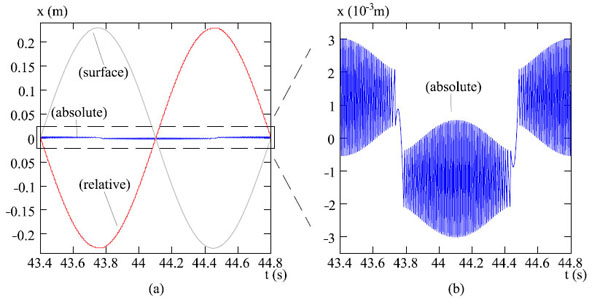) shows a plot of the block’s absolute displacement, together with that relative to the surface, during one period. High-frequency oscillations superposed on that of the surface are clearly identifiable in Fig. (6b), which shows an enlargement of the block’s absolute displacement. The diagram of the absolute velocity of the block and surface, plotted in Fig. (7a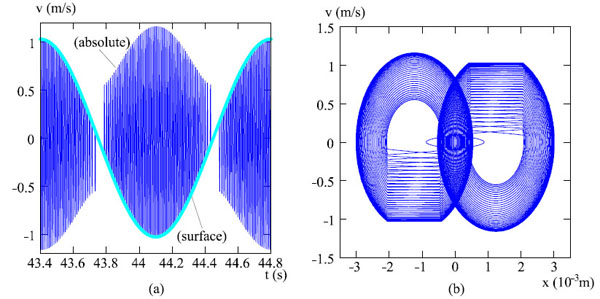), as well as the phase diagram shown in Fig. (7b), reveal that the block’s motion tends to become periodic after an initial transient phase. In particular, a high-frequency stick-slip alternation with slowly varying amplitude is observed. The numerical results are fully consistent with the theoretical predictions represented in the diagrams plotted in Fig. (4).

The frequency content of signal x(t), illustrated in Fig. (8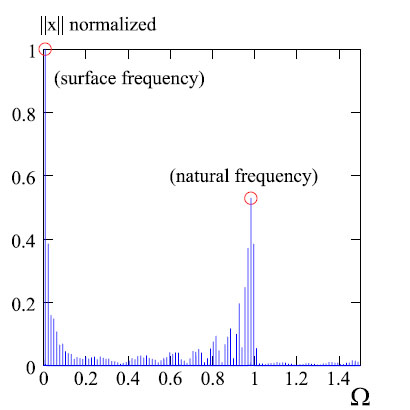), clearly shows that the dominant frequency nearly coincides with that of the oscillations of the moving surface. Together with many minor peaks, a second major peak in the diagram corresponds to the natural frequency of the mass-spring system. These peaks can be explained by observing that the frequency of the alternations of the stick and slip phases varies over a single period of the surface’s oscillation. The signal x(t), properly scaled, also represents that of the reactive elastic force. Recalling that, in our scheme, this force corresponds to that exchanged between the rubber lip and the vertebra, these results seem to suggest that high-frequency vibrations are likely to be transmitted to the vertebra and the other components of the wiper system as well. Such vibrations may in some cases lead to the onset of the dynamic instabilities (such as chattering or fishtailing) that are sometimes observed in wiper systems. On the other hand, note that the frequency content of the contact force exchanged between the block and the surface (i.e., the rubber lip and the screen, in our scheme) contains but a single peak in correspondence to the frequency of the surface’s oscillations.Fig. (5)Sketch of a typical portion of the block’s motion.Fig. (6)(a) Absolute and relative position of the block, absolute position of the surface; (b) absolute position of the block (magnified 1000 times).Fig. (7)(a) Absolute velocity of the block and velocity of the surface. (b) Phase trajectory of the block in the (x,ẋ) plane.Fig. (8)Frequency spectrum of signal x(t).

## CONCLUSION

In this paper we have studied the dynamic response of wiper systems viewed as an assemblage of elements that can slide one over the other in the presence of significant friction. In particular, we have studied a first elementary model in which a rigid block connected to an elastic spring is free to slide over a rough surface moving according to a sinusoidal law of motion. The contact between the block and the surface is described by a suitably modified version of Coulomb’s law. The modification enables accounting for the short time usually needed to restore static contact condition after a sliding phase.

Although the system dynamics proves to be highly nonlinear overall, due to the nonlinear friction law used, the equations of motion for the block can be easily solved in each phase. The exact sequence of sticking and sliding phases, starting with given initial conditions, can be predicted by means of two simple criteria that allow for determining the onset or the end time of a sliding phase, and the sign of the friction force at the beginning of a sliding phase as well. The results obtained for an application case concerning a wiper blade enable highlighting the high-frequency stick-slip motion of the rubber lip together with the frequency content of the forces exchanged between the rubber lip and the vertebra, as well as between the rubber lip and the screen.

We have moreover discussed the influence on the solution of three dimensionless parameters chosen to characterize the system: the ratio Ω between the surface oscillation frequency and the system’s natural frequency, the ratio η between the kinetic and the static friction coefficients, and the ratio α between the maximum surface oscillation amplitude and the maximum distance of the block from the origin, which corresponds to it being in a state of static equilibrium.

Some typical limit cycles corresponding to continuous sliding or sticking have then been analyzed. In particular, we have detailed the conditions necessary to ensure the existence of such limit cycles, and analyzed some of the limit cycles that can actually be attained by the system. We have shown that, for given η, the (Ω, α) plane can be partitioned into different regions, each corresponding to a different dynamic system response. According to the results, once the main system parameters α, Ω and η are fixed, it is possible to foresee some basic features of its possible long-term responses. In particular, we have determined the cases for which continuous sticking (or, alternatively, period-one sliding) can be excluded, and, instead, those for which only steady stick-slip motions are to be expected.

The analysis of stick-slip systems performed by directly accounting for the moving surface, as described herein, seems to be an effective strategy for achieving a better understanding of the main features of the dynamic response of such systems. Such a modeling strategy may also represent a rational framework for enabling more direct interpretation of the results than that obtainable by means of the alternative approach (i.e., by considering a harmonically loaded system).

## APPENDIX

### The Sticking End Time Criterion

The criterion adopted for determining the sticking end time may be explained by means of the diagrams plotted in Fig. (9). We refer, for the sake of simplicity, to the case where λ > 0 and the sticking start time is zero.

The sticking phase ends when one of the two equalities (12a) or (12b) becomes true. Graphically, such criterion corresponds to determining the (first) instant at which the plot of the function of time α(1 - Ω2)sinωbt + λ exits from the strip bounded by the straight lines with ordinates ±1.

Basically, two cases must be considered depending on whether or not α |1 - Ω2| is smaller than 1 + λ. In the first case, by looking at the left-hand diagram of Fig. (9), it is straightforward to conclude that within a single period there is only one possibility left (the colored circle labeled A on the diagram), and the end time is determined according to (12a). On the other hand, in the second case, two points meet the requirements (B and C, right hand of the figure). The choice between the two is made according to the sign of the first derivative (i.e., the sign of (1 - Ω2)cosωbt). The correct choice will be point B or C (i.e., the solution to (12a) or (12b), depending on whether said sign is positive or negative, respectively. Fully analogous considerations apply to the other cases listed in the criterion in section 3.1.Fig. (9)The sticking end time criterion.

### CONFLICT OF INTEREST

The authors confirm that this article content has no conflict of interest.

## ACKNOWLEDGEMENTS

The financial support of Robert Bosch Produktie NV is gratefully acknowledged.

## REFERENCES

  C. Chevennement-Roux, T. Dreher, P. Alliot, E. Aubry, J-P. Lainé, and L. Jézéquel, "Flexible wiper system dynamic instabilities: modelling and experimental validation", Exp. Mech., vol. 47, pp. 201-210, 2007.[http://dx.doi.org/10.1007/s11340-006-9027-3]  R. Suzuki, and K. Yasuda, "Analysis of chatter vibration in an automotive wiper assembly", JSME Int. J. Ser. C, vol. 41, no. 3, pp. 616-620, 1998.[http://dx.doi.org/10.1299/jsmec.41.616]  G. Lancioni, S. Lenci, and U. Galvanetto, "Non-linear dynamics of a mechanical system with a frictional unilateral constraint", Int. J. Non-linear Mech., vol. 44, no. 6, pp. 658-674, 2009.[http://dx.doi.org/10.1016/j.ijnonlinmec.2009.02.012]  J. Den Hartog, "Forced vibrations with combined coulomb and viscous friction", Trans. ASME, vol. 53, no. 9, pp. 107-115, 1931.  R. Parnes, "Response of an oscillator to a ground motion with coulomb friction slippage", J. Sound Vibrat., vol. 94, no. 4, pp. 469-482, 1984.  E. Marui, and S. Kato, "Forced vibration of base-excited single-degree-of-freedom system with coulomb friction", J. Dyn. Syst. Meas. Control, vol. 106, no. 4, pp. 280-285, 1984.[http://dx.doi.org/10.1115/1.3140686]  S.W. Shaw, "On the dynamic response of a system with dry friction", J. Sound Vibrat., vol. 108, no. 2, pp. 305-325, 1986.[http://dx.doi.org/10.1016/S0022-460X(86)80058-X]  H-K. Hong, and C-S. Liu, "Non-sticking oscillation formulae for coulomb friction under harmonic loading", J. Sound Vibrat., vol. 244, pp. 883-898, 2001.[http://dx.doi.org/10.1006/jsvi.2001.3519]  V. Andreaus, and P. Casini, "Dynamics of friction oscillators excited by a moving base and/or driving force", J. Sound Vibrat., vol. 245, no. 4, pp. 685-699, 2001.[http://dx.doi.org/10.1006/jsvi.2000.3555]  J.M. Gibert, G. Fadel, and M.F. Daqaq, "On the stick-slip dynamics in ultrasonic additive manufacturing", J. Sound Vibrat., vol. 332, no. 19, pp. 4680-4695, 2013.[http://dx.doi.org/10.1016/j.jsv.2013.04.007]  E.I. Butikov, "Spring pendulum with dry and viscous damping", Commun. Nonlinear Sci. Numer. Simul., vol. 20, no. 1, pp. 298-315, 2015.[http://dx.doi.org/10.1016/j.cnsns.2014.04.026]  R. Barsotti, S. Bennati, and F. Quattrone, "Dyanamical response of a single degree of freedom system in contact with a rough moving surface. (in Italian)", G. Lacidogna, and A. Carpinteri, Eds., Proceedings of AIMETA, 2013  J. Sampson, F. Morgan, D.W. Reed, and M. Muskat, "Friction behavior during the slip portion of the stick-slip process", J. Appl. Phys., vol. 14, no. 12, pp. 689-700, 1943.[http://dx.doi.org/10.1063/1.1714948]  G. Csernák, and G. Stépán, "On the periodic response of a harmonically excited dry friction oscillator", J. Sound Vibrat., vol. 295, no. 3-5, pp. 649-658, 2006.[http://dx.doi.org/10.1016/j.jsv.2006.01.030]  S. Chatterjee, "Resonant locking in viscous and dry friction damper kinematically driving mechanical oscillators", J. Sound Vibrat., vol. 332, no. 14, pp. 3499-3516, 2013.[http://dx.doi.org/10.1016/j.jsv.2012.12.042]  G. Licskó, and G. Csernák, "On the chaotic behaviour of a simple dry-friction oscillator", Math. Comput. Simul., vol. 95, pp. 55-62, 2014.[http://dx.doi.org/10.1016/j.matcom.2013.03.002]  M. Pascal, "New limit cycles of dry friction oscillators under harmonic load", Nonlinear Dyn., vol. 70, no. 2, pp. 1435-1443, 2012.[http://dx.doi.org/10.1007/s11071-012-0545-5]  E. Rabinowicz, "The intrinsic variables a_ecting the stick-slip process", Proc. Phys. Soc., vol. 71, no. 4, pp. 668-675, 1958.[http://dx.doi.org/10.1088/0370-1328/71/4/316]  R.I. Leine, D.H. Van Campen, and A. De Kraker, "Stick-slip vibrations induced by alternate friction models", Nonlinear Dyn., vol. 16, pp. 41-54, 1998.[http://dx.doi.org/10.1023/A:1008289604683]  G.J. Stein, R. Zahoranský, and P. Múčka, "On dry friction modelling and simulation in kinematically excited oscillatory systems", J. Sound Vibrat., vol. 311, pp. 74-96, 2008.[http://dx.doi.org/10.1016/j.jsv.2007.08.017]  G. Bódai, and T.J. Goda, "Sliding friction of wiper blade: Measurement, FE modeling and mixed friction simulation", Tribol. Int., vol. 70, pp. 63-74, 2014.[http://dx.doi.org/10.1016/j.triboint.2013.07.013]

### Endorsements

"Open access will revolutionize 21st century knowledge work and accelerate the diffusion of ideas and evidence that support just in time learning and the evolution of thinking in a number of disciplines."

Daniel Pesut
(Indiana University School of Nursing, USA)

"It is important that students and researchers from all over the world can have easy access to relevant, high-standard and timely scientific information. This is exactly what Open Access Journals provide and this is the reason why I support this endeavor."

Jacques Descotes
(Centre Antipoison-Centre de Pharmacovigilance, France)

"Publishing research articles is the key for future scientific progress. Open Access publishing is therefore of utmost importance for wider dissemination of information, and will help serving the best interest of the scientific community."

Patrice Talaga
(UCB S.A., Belgium)

"Open access journals are a novel concept in the medical literature. They offer accessible information to a wide variety of individuals, including physicians, medical students, clinical investigators, and the general public. They are an outstanding source of medical and scientific information."

Jeffrey M. Weinberg
(St. Luke's-Roosevelt Hospital Center, USA)

"Open access journals are extremely useful for graduate students, investigators and all other interested persons to read important scientific articles and subscribe scientific journals. Indeed, the research articles span a wide range of area and of high quality. This is specially a must for researchers belonging to institutions with limited library facility and funding to subscribe scientific journals."

Debomoy K. Lahiri
(Indiana University School of Medicine, USA)

"Open access journals represent a major break-through in publishing. They provide easy access to the latest research on a wide variety of issues. Relevant and timely articles are made available in a fraction of the time taken by more conventional publishers. Articles are of uniformly high quality and written by the world's leading authorities."

Robert Looney

"Open access journals have transformed the way scientific data is published and disseminated: particularly, whilst ensuring a high quality standard and transparency in the editorial process, they have increased the access to the scientific literature by those researchers that have limited library support or that are working on small budgets."

Richard Reithinger
(Westat, USA)

"Not only do open access journals greatly improve the access to high quality information for scientists in the developing world, it also provides extra exposure for our papers."

J. Ferwerda
(University of Oxford, UK)

"Open Access 'Chemistry' Journals allow the dissemination of knowledge at your finger tips without paying for the scientific content."

Sean L. Kitson
(Almac Sciences, Northern Ireland)

"In principle, all scientific journals should have open access, as should be science itself. Open access journals are very helpful for students, researchers and the general public including people from institutions which do not have library or cannot afford to subscribe scientific journals. The articles are high standard and cover a wide area."

Hubert Wolterbeek
(Delft University of Technology, The Netherlands)

"The widest possible diffusion of information is critical for the advancement of science. In this perspective, open access journals are instrumental in fostering researches and achievements."

Alessandro Laviano
(Sapienza - University of Rome, Italy)

"Open access journals are very useful for all scientists as they can have quick information in the different fields of science."

Philippe Hernigou
(Paris University, France)

"There are many scientists who can not afford the rather expensive subscriptions to scientific journals. Open access journals offer a good alternative for free access to good quality scientific information."

Fidel Toldrá
(Instituto de Agroquimica y Tecnologia de Alimentos, Spain)

"Open access journals have become a fundamental tool for students, researchers, patients and the general public. Many people from institutions which do not have library or cannot afford to subscribe scientific journals benefit of them on a daily basis. The articles are among the best and cover most scientific areas."

M. Bendandi
(University Clinic of Navarre, Spain)

"These journals provide researchers with a platform for rapid, open access scientific communication. The articles are of high quality and broad scope."

Peter Chiba
(University of Vienna, Austria)

"Open access journals are probably one of the most important contributions to promote and diffuse science worldwide."

Jaime Sampaio
(University of Trás-os-Montes e Alto Douro, Portugal)

"Open access journals make up a new and rather revolutionary way to scientific publication. This option opens several quite interesting possibilities to disseminate openly and freely new knowledge and even to facilitate interpersonal communication among scientists."

Eduardo A. Castro
(INIFTA, Argentina)

"Open access journals are freely available online throughout the world, for you to read, download, copy, distribute, and use. The articles published in the open access journals are high quality and cover a wide range of fields."

Kenji Hashimoto
(Chiba University, Japan)

"Open Access journals offer an innovative and efficient way of publication for academics and professionals in a wide range of disciplines. The papers published are of high quality after rigorous peer review and they are Indexed in: major international databases. I read Open Access journals to keep abreast of the recent development in my field of study."

Daniel Shek
(Chinese University of Hong Kong, Hong Kong)

"It is a modern trend for publishers to establish open access journals. Researchers, faculty members, and students will be greatly benefited by the new journals of Bentham Science Publishers Ltd. in this category."

Jih Ru Hwu
(National Central University, Taiwan)

Webmaster Contact: info@benthamopen.net